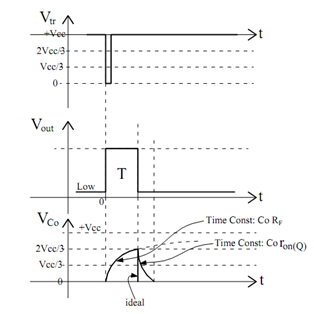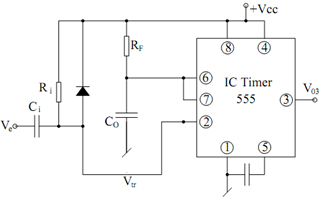## Various Waveforms of the Monostable Multivibrator Assignment Help

Assignment Help: >> Monostable Multivibrator - Various Waveforms of the Monostable Multivibrator

Various Waveforms of the Monostable Multivibrator:

Now since, V2 < 1/3 Vcc, pin 7 opens up and capacitor C0 charges exponentially towards + Vcc and continues till Vco >(2/3) Vcc at which time, timer output goes LOW. Now, pin 7 is shorted to ground and Vco becomes zero. Therefore, the circuit comes back to its permanent stable state and shall remain so unless another trigger pulse is applied to begin the operation all over again.Figure: Various Waveforms of the Monostable Multivibrator

The time period during which waveform remains HIGH can be determined as follows.

The general equation for the capacitor voltage may be written as Vco = A + B e -t/R f C0   in

which constants A and B may be found by applying the conditions : at t = 0 , Vco = 0 and at t = ∞ , Vco = + Vcc which provides the values of A and B as A = Vcc and B = - Vcc, so that

we have,

Vco  = Vcc  (1 - e -t/R f C0  )

Now for t = T, Vco =  +(2/3 )Vcc, therefore, one finds,

T = RF C0  ln 3

The required trigger pulse may be obtained by the modification illustrated in Figure which is self-explanatory.Figure: Modified Monostable Multivibrator